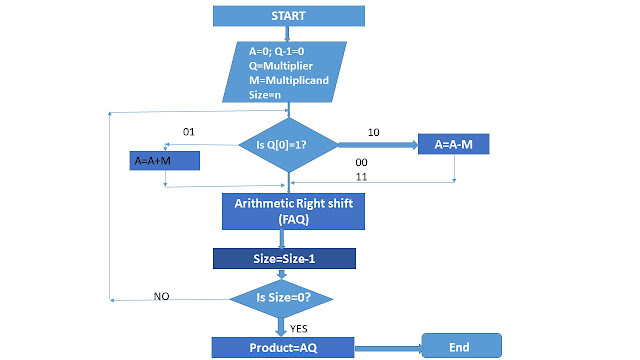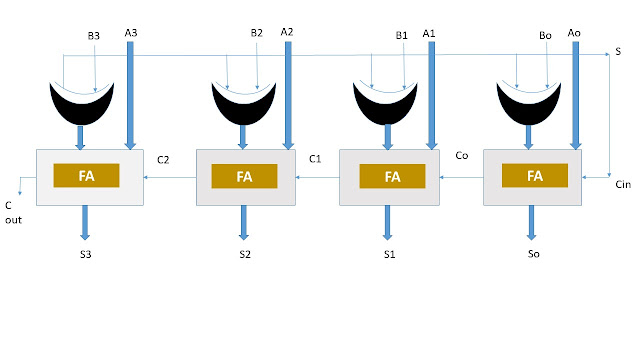## Sunday, 17 February 2019

Module II

### SEQUENTIAL MULTIPLICATION METHOD FOR UNSIGNED NUMBERS::

• #### This multiplication method is described in the flow chart as shownfig: Sequential Multiplication Method

Here, the register A is called accumulater register,  initialized to 0. f is the flip-foops which holds
generated in the end carry in the addition,
*f is used as the serial input, when the register.pair AQ is shifted right one position, sequential position.

## Q. Perform the equential multiplication for 6*14 for n=4

 Initial configuration F A Q 0 0000 1110 SIZE=4 0 0 0000 0000 1110 0111 Q=0 Arithamectic Shift Right FAQ SIZE=3 0 0 0110 0011 0111 0011 Q0=1,A=A+M Arithamectic Right FAQ SIZE=2 0 0 1001 0100 0011 1001 Q0=1,A=A+M Right Shift  FAQ SIZE=1 0 0 1010 0101 1001 0100 Q0=1,A=A+M Right Shift FAQ SIZE=0

product = AQ = 01010100 = 84 Ans.

## Q) Perferm sequential multiplication mothered for unsigned number - a) 7 X6 (6) 11 X20 (c) 6x5

Booth's multiplication Procedure (for signed number):

1) Booth multiplication method, introduce a new bit  Q-1 which is initialised by zero.
2)This method uses 2’s complement method.
3)2’s  complement A positive number as same as their binary representation.
Advantages of Booths multiplication:
1) Pre-processing steps are unnecessary,so the Booth's algorithm treats signed number in a uniform way with unsigned number.
2)Less number of addition and subtraction are required, compared to the sequential multiplication method..

A   Complement representation of Q=>0111 2's complement representation of M => +6=1001(1’s                complement)=1010(-6)
M= 1010)

 A Q Q-1 Size 0000 0111 0 0110 0011 0111 0011 0 1 (Q)_0*Q_1=10 A=A-M Arithematic right shift AQQ_1 Size=3 0001 1001 1 (Q)_0*Q_1=11 A=A-M Arithematic shift right AQQ_1 Size=2 0000 1100 1 (Q)_0*Q_1=11 A=A-M Arithematic shift right AQQ_1 Size=1 1010 1101 1100 0110 1 1 (Q)_0*Q_1=01 A=A-M Arithematic shift right AQQ_1 Size=0
Poduct = AQ = 11010110 in 2's complement form
* To get number in familias form, take the 2's
complement of the magnitude.
00101001
+1

00101010 (-42),. Ang

### Any division is related as

D=>QV+R  (Where 0<=R<V)
D=>Dividend
V=>Divisor
R=>Remainder
Q=> Quotient

#### Restoring  Division Method:

fig: Restoring Division Algorithm

* In this method, instead of shifting the divisor, shift the dividend to the left
* This method use three n-bit registers A,M and Q . for dividing two n-bit numbers.

Example
consider the example    7/3
Dividend Q = 7 (0111),
Divisor M= 3 (0011)
M: 0011

 A Q Size 0000 111- 0000 1101 0000 111- 111- 1110 Left shift AQ A=A-M A<0,Qo=0 A=A+M 3 0001 1110 0001 110- 110- 1100 Left shift AQ A=A-M A<0,Qo=0 A=A+M 2 0011 0000 0000 100- 100- 1001 Left shift AQ A=A-M A=0,Qo=1 1 0001 1110 0001 001- 001- 0010 Left shift AQ A=A-M A<0,Qo=0 A=A+M 0

Ans      remainders (A)=1 (0001)

Quoteint = Q=2 (0010).

## Non- restering Division Method:

* In restoring method, some extra additions are required when A is negative.
* Proper restructuring of the restoring division algorithm can eliminate that restoration step. "This is known as the non-restoring division method.

perform 7/3 by non restoring division

Divisor (M)= 0011

Accemulater (A)     Dividend(Q)

 A Q Size 0000 0111 0000 1101 1101 111- 111- 1110 A=0, Left Shift AQ A<0,Qo=0 3 1011 1110 1110 110- 110- 1100 A<0,Left shift AQ A=A+M A<0,Qo=0 2 1101 0000 0000 100- 100- 1001 Left shift AQ,A

## DESIGN OF ADDER

Binary Adder: -
* The microtoperations, additions and subtraction of two binary numbers stored in two registers are performed by binary adder/sus practor.
*A binary adder is a digital circuit that generates the arithmetic sum of two binary numbers of any length.

* Binary adder is basically construction acted with full addess.
* Bincity adder is of two types - parallel adder and serial adder.

Parallel Adder:
*A parallel adder is adder, which add all bits of two numbers.
*It has separate adder circuit for each bit. Therefore, to add two . n-bit numbers, parallel adder needs in separate adder circuits.
* There are basically two types of parallel adder, depending on the way of carry generation
(i) Carry-Propagate Addeyr (CPA) or Ripple Carry Adder (RCA) Cl)
(ii) Carry-Look-ahead addex (CLA).

a) Carry Propagate Array (CPA):

* for addition of two n-bit numbers, in full adders are required.
* Each full adder's carry output will be the input of the next higher bit adder.
* Each fall adder performs addition for same position bits of two numbers.
* An n-bit CPA circuit is as shown.
* In worst case, the carry from the first full adder stage has to propagate through all the full adder stage.
* Therefore, the maximum propagate delcey for n-bit CPA is sxn , where o is the time delay for each full adder. stage and n is the numbers of bits in each operand.
Advantage:
This circuit, being a combinational circuit, is faster than serial adder.
Disadvantages

1) The addition delay becomes large, if the size of numbers to be added is increased.
2 The hardware cast is more than that of senal adder. Because, the number of full adders needed is equal to the number of bits in operands.

b) Carry Look Ahead (CLA) :

*A CLA is a high speed adder, which adds two numbers without waiting for the carries from the previous stages.
* In CLA, carry inputs of all stages are generated simultanerisly, without using carries from the previous stages.
* Ci+1 is related to Ci as

Ci+1= Ai Bi + (Ai +Bi) Ci]
»Ci+1=Gi+PiCi
Gi is called carry generator & Pi is called carry propagate.

* Now, we want to design a 4-bit CLA for which four carries C1,C2, C3 and C4 are to be generated,

so,
C1= G0+PoCo
C2= G1+ P1 C1
C3= G2+P2 C2
C4 = G3+ P3 C3
These equations are recursive and the recursion can be removed as

C1= Go+ PoCo
C2= G1+ P1C1
C2= G1+ P1 (Go + PoCo).
C2= Gi+ P1Go+P1PoCo
C3= G2 P2C2
=G2+P2(G1+P1Go+P1PoCo(0)
=G2+P2G1+P2P1Go+P2P1PoCo
C4=G3+P3C3
=G3+P3G2+P3P2G1+P3P2P1Go+P3P2P1Go+P3P2P1Po

Get Pz (Git P, Got Pipoco).

= Gat P2 GL + P2 Pj Got P2P, polo (4= GB + PB C3 Co

= Gg+ P3 G 2 + P3P 2G jt PB P2P, Got P3 Pzp, Polo

fig 4-bit carry, look ahead Adder.

Binary.Adder- Subpractor unit:* The addition and subtraction can be combined to a single circuit by using exclusive-OR (XOR) gate with each full adder.
* This is as shown.
*s is the selection input.
* when s is o then this circuit perform binery addition AS OB B-B.
* When sis 1, then this circuit perform binary subtraction, As 1 + B = B
DESIGN of ALU
* In design of ALU, following three units are used:

a) Arithmetic unitin

* Diagram of a 4- bit, arithmetic circuit is shown is the diagram
* The circuit has a 4-bit paralled adder and four multiplexers for 4-bit arithmetic unit.
* There are two 4-bit inputs A and B and the 5 bit output is k.
* The size of each multiplexer is 4:1.

* So & S1 are selection lines.
Fig: 4-bit Arithmetic Unit

Here K=A+ Cin. Arithmetic unit function table is given as-

 S1 S0 Cin Y K=A+Y+Cin Operation 0 0 0 B K=A+B Addition 0 0 1 B K=A+B+1 Addition with carry 0 1 0 B(BAR) K=A+B(BAR) Subtraction with borrow 0 1 1 B(BAR) K=A+B(BAR)+1 Subtraction 1 0 0 1 K=A-1 Decrement 1 0 1 1 K=A Transfer 1 1 0 0 K=A Transfer 1 1 1 1 K=A+1 Increment

In the above unit we have four cases:-
cased when S1So =00

In this case the values of B is selected to the Ý inputs of the adder
If Cin=0; output K=A+B

If Cin= 1, output K = A + B +1

case 2: when S1,So = 01

B is selected to y inputs of the adder
If Cin=0, K= A + B(BAR)

IF Cin=1; K= A + B(BAR)+1
= Ke A+ 2's complement of B
coese 3: when Seso=10.

All 1's are selected to the y inputs of the adder means Y=(1111), which is equivalent to the 2's complement of decimal 1, Eg. Y= -1

If Cin=0, K= A-1 ( decrement operation )
If Cin= 1, K= A-1+1= A ( transfer of A to k)

Case4 when S1 So=11.

All 0's are selected to the Y input of addere,

If Cin=0, K= A,
If Cin=1, K=A+1:

- *Logic unit is needed to perform micro-operations such as OR, AND, XOR, complement, on individual pairs of bits stored in registers.
Eg:  C:RI<- R2 V R3

The OR(V) micro-operation between the contents of two registers R2 and R3 can be stated as above.
* For AND micro-operation, the used symbol is ^
* For XOR micro-operation, the used symbol is +
* For Not (or complement) micro-operation, the used symbol is - (bar):
One,ith  stage logic unit for thesebfour basic micro-operations is shown as

fig: one.ith stage logic unit
* To design a 4-bit logic unit four 4:1 multiplexers and . 16 gates are required.

 S1 S0 OUTPUT(L) OPERATION 0 0 L=A^B AND 0 1 L=AvB OR 1 0 L=A+B XOR 1 1 L=A(BAR) COMPLEMENT OF A

Shifter Unit:

* Shifter unit is used to perform shift - micro-operations,
* Shift- micro-operations are used to transfer stored data Serially.
* The shifting of bits of a register can be in either direction left or right.
* Shift micro-operations can be classified into three categories - Logical, circular and Arithmetic.
a) Logical shift :

All bits including sign bit, take part in the shift oporation
A bit 0 is entered in the vacant extreme : bit position (left mest or right most).

b Circular Shift:
In circular shist (also known as rotation operation), one bit shitted out from one extreme bit position ; enters the other. extreme side's vacant bit position as shown

e) Arithmetic shift:

In this shift, sign bit remains unaffected and other bits (magnitude bits) take part in shift micro -operation as shown

" Shifter unit can be constructed using bidirectional

shift- register using clock circuit.
* But, a combinational shifter unit can be constructed with multiplexers as shown

*Ao,A1 and A2 are three data input data output are GO,G1,G2.
There are two serial input I2(left shift operation) and Ir(right shift operator)
if S is 0, the stored input data is shifted right
if S is 1, the stored input data is shifted left

 Slectline Output

 S Go G1 G2 0 IR Ao A1 1 A1 A2 I
Now we can design ALU with common select lines
Here we design a 4-bit 14 function ALU

 S3 S2 S1 So Cin OUTPUT OPERATION 0 0 0 0 0 F=A+B Addition 0 0 0 0 1 F=A+B+1 Addition with carry 0 0 0 1 0 F=A+B(BAR) Substraction with borrow 0 0 0 1 1 F=A+B(BAR)+1 Substraction 0 0 1 0 0 F=A-1 Decrement A 0 0 1 0 1 F=A Transfer 0 0 1 1 0 F=A Transfer 0 0 1 1 1 F=A+1 Increment 0 1 0 0 X F=A^B AND 0 1 0 1 X F=AvB OR 0 1 1 0 X F=A+B X-OR 0 1 1 1 X F=A(BAR) Complement of A 1 0 0 X X F=Lgr A Left shift right A into F 1 0 1 X X F=Lsl A Shift left A into F

#### 1 comment:

1.such a nice artical...
https://technologytipsfaizi.com

### Basic Electronics Engineering (EC101)

Basic Electronics Engineering (EC101)